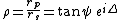# ellipsometry

Also found in: Wikipedia.

## Ellipsometry

A technique for determining the properties of a material from the characteristics of light reflected from its surface. The materials studied include thin films, semiconductors, metals, and liquids.

When an electromagnetic wave passes through a medium, it causes the electrons associated with the atoms of the medium to oscillate at the frequency of the wave. As a result, the wave is slowed so that its velocity in the medium is less than its velocity in empty space. Another result may be a transfer of energy from the wave to the electrons, thereby causing the amplitude of the wave to decrease as it penetrates into the material. These two processes are described phenomenologically by the complex refractive index. See Absorption of electromagnetic radiation, Refraction of waves

When an electromagnetic wave is incident on a medium, only part of it is transmitted into the medium. The fraction reflected depends on the complex refractive index, the angle of incidence, and the polarization state of the wave. For multilayers with different complex refractive indices, the fraction also depends on the layer thicknesses. The two basic types of polarization are parallel, designated p, and perpendicular, designated s. These terms refer to the orientation of the electric vector with respect to the plane of incidence, which is defined by the directions of the incident and reflected waves. The (intensity-independent) ratios of the amplitudes and phases of the reflected and incident p- and s-polarized electric fields are described by the complex reflectances rp and rs. See Polarization of waves, Polarized light, Reflection of electromagnetic radiation

The (also intensity-independent) ratio of the p- to the s-polarized component of such a wave is termed the polarization state. Simple examples include linear polarization, where the p- and s-polarized components are in phase, and circular polarization, where the p- and s-polarized amplitudes are equal but the phases differ by 90°. The geometric terms refer to the locus of the p and s (or y and x) components of the electric field when plotted in the complex plane. The general polarization state is elliptical.

Since the complex reflectances rp and rs depend on the properties of the medium, the medium can be investigated by determining its reflectance for either p- or s-polarized light, that is, by determining the ratio of reflected and incident intensities Irefl/Iinc = R = . This is the objective of reflectometry. Alternatively, because rp and rs are different, the complex reflectance ratio &rgr; = rp/rs, which is equal to the ratio of reflected and incident polarization states, can also be determined. This is the objective of ellipsometry. The ratio &rgr; is traditionally expressed in terms of angles &psgr; and Δ as in the equation below.Because it deals with complex, intensity-independent quantities, an ellipsometric measurement is analogous to an impedance measurement. This gives ellipsometry certain advantages relative to reflectometry, such as higher accuracy and higher information content in a single measurement. A standard experimental approach, now used almost exclusively in spectroscopic applications, is to determine &rgr; by establishing a known state of polarization for the incident beam, for example, by passing it through a fixed linear polarizer, then determining the polarization state of the beam after reflection by passing it through a rotating linear polarizer, called an analyzer. The rotating analyzer essentially unrolls the polarization ellipse, allowing the azimuth angle of its major axis and its minor-major axis ratio to be determined by the phase and amplitude of the alternating-current component of the detected intensity. See Electrical impedance

The primary application of ellipsometry is materials analysis, particularly the nondestructive analysis of thin films in semiconductor technology. Developing applications include the real-time monitoring and control of dynamic processes such as material deposition and etching. Spectroellipsometry, where the complex refractive index is measured and analyzed as a function of wavelength, has almost exclusively replaced reflectometry in materials analysis. Deposition and etching involve kinetic ellipsometry, where single-wavelength data are monitored as a function of time. See Semiconductor heterostructures

McGraw-Hill Concise Encyclopedia of Physics. © 2002 by The McGraw-Hill Companies, Inc.

## ellipsometry

[ə‚lip′säm·ə·trē]
(optics)
A technique for determining the properties of a material from the polarization characteristics of linearly polarized incident light reflected from its surface.
McGraw-Hill Dictionary of Scientific & Technical Terms, 6E, Copyright © 2003 by The McGraw-Hill Companies, Inc.
References in periodicals archive ?
Various techniques, including neutron reflectometry [2, 3], ellipsometry [4-6], secondary-ion mass spectrometry , Raman spectroscopy , infrared spectroscopy , X-ray reflectometry , and energy-filtering transmission electron microscopy [11, 12], have been used to analyze the interfacial behavior in polymer blends.
Ellipsometry from infrared to vacuum ultraviolet: structural properties of thin anisotropic guanine films on silicon.
Goullet, "In situ spectroscopic ellipsometry study of Ti[O.sub.2] films deposited by plasma enhanced chemical vapour deposition," Applied Surface Science, vol.
The characteristics of optical absorption and polarization of the ZnO NR were investigated by spectroscopic ellipsometry Micropack: Spec-EI 2000.
In this study, we focus on the growth process of CIGS films for various thicknesses (1.95 [micro]m to 0.35 pm) characterized by in situ real-time spectroscopic ellipsometry (RTSE), and the results are correlated with ex situ measurements such as XRF, XRD, and SEM.
The thickness of [Al.sub.2][O.sub.3] and Al-ZrHf[O.sub.2] was the same as 30 nm, which was confirmed by ellipsometry measurement.
Schienle et al., "In situ monitoring of InAs-on-GaAs quantum dot formation in MOVPE by reflectance-anisotropy-spectroscopy and ellipsometry," Applied Surface Science, vol.
Ellipsometry measures the two values [psi] and [DELTA], which express the amplitude ratio and phase difference between p polarization and s polarization, shown in (1).
The thickness and the optical constants of Ti[O.sub.2] thin films fabricated at different deposition times were determined by spectroscopic ellipsometry. The measurements were carried out using a variable-angle ellipsometer (M-2000; J.A.
Azpuru, "The electrochemical behaviour of copper in alkaline solutions containing fluoride, studied by in situ ellipsometry," Surface Science, vol.
Chen, "Using spectroscopic ellipsometry to characterize and apply the optical constants of hollow gold nanoparticles," ACS Nano, vol.
Site: Follow: Share:
Open / Close